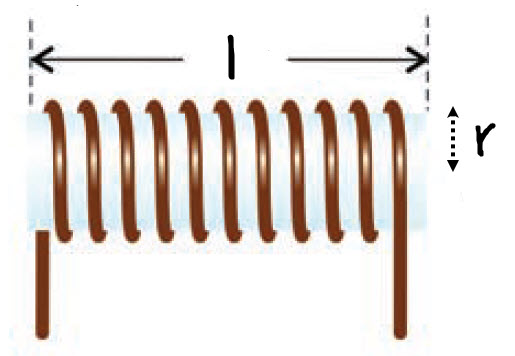# Air Core Inductor Calculator

Below is online calculator for calculating number of turns or inductance required to make air core inductor.

Calculate N:

cm
cm

Result:
mm

Calculate L:
cm
cm

Result:
µH mmThe formula for such single layer air core inductor is given below.

$$L = \frac{0.394 r^2 N^2}{9r+10l}$$

where,

- L is inductance in uH

- r is the radius in cm

- l is length in cm

To use this formula we must have r > 0.67l.

Example:

Let us calculate the number of turns(N) required for make 22uH inductor. Let the length of the inductor be 2cm and radius(r) be 1cm. Then using the above formula we get the number of turns N =40.24 which can be taken approximately equal to 4.

To select which AWG(American Wire Gauge) number/size to use we have to calculate the diameter of the wire required. After knowing the diameter, we can use AWG wire table and choose AWG number. The required wire diameter can be calculated using the following formula.

$D = \frac{l}{N}$

Using this value of diameter(D) we can select the appropriate AWG number from the following table.

In this example above the diameter(D) of the wire is 0.5mm. From the AWG table the closest AWG wire size is AWG 24 which has diameter of 0.51mm. To reduce the capacitance that may develop between the wires some spacing is desirable. So selecting the next AWG will more diameter is 23 which should be selected.

Related Topics

A rough guidance of making coil to operate the inductor in the frequency range 50MHz to 150MHz, with number of turns and radius is shown below.